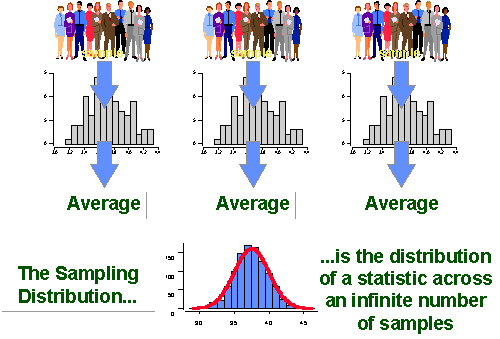# Sampling Distributions Assessment Test

10 Questions | Total Attempts: 161SettingsIn general, a sampling distribution is a probability distribution of a statistic obtained through a large number of samples drawn from a specific population. Take this assessment test to find out more.

• 1.
A probability distribution of a statistic obtained through a large number of samples drawn from a specific population is...
• A.

Graph

• B.

Sampling distribution

• C.

Sample

• D.

Table

• 2.
The actual or estimated standard deviation of the error in the process by which it was generated is...
• A.

Open error

• B.

Standard error

• C.

Closed error

• D.

Real value

• 3.
The statistical information from a sample which will vary as the random sampling is repeated is referred to as...
• A.

Linear

• B.

Nominal

• C.

Sampling variability

• D.

Coefficient

• 4.
A listing or function showing all the possible values of data and how often they occur is...
• A.

Table

• B.

Distribution

• C.

Arrangement

• D.

Line

• 5.
Randomly choosing a sample of k items from a list S containing n items, where n is either a very large or unknown number is...
• A.

Reservoir sampling

• B.

Rule sampling

• C.

Nomination

• D.

Ordination

• 6.
A statistical tool for constructing sampling distributions is the...
• A.

Permutation test

• B.

Combination test

• C.

T test

• D.

Confidence test

• 7.
A statistical test used on paired nominal data is the...
• A.

McNemar's test

• B.

Apte test

• C.

F test

• D.

Linear test

• 8.
What is used to test the null hypothesis that the median of a distribution is equal to some value?
• A.

Lean test

• B.

Poll test

• C.

Sign test

• D.

Pill test

• 9.
Which is a method for testing differences between three or more matched sets of frequencies or proportions?
• A.

T test

• B.

Optimum test

• C.

Cochran's Q test

• D.

D test

• 10.
What is used for identification and rejection of outliers?
• A.

A test

• B.

E test

• C.

T test

• D.

Q test

Related TopicsBack to top